Publicité

# Depreciation and the Time Value of Money: A primer of the arXiv article

13 Dec 2016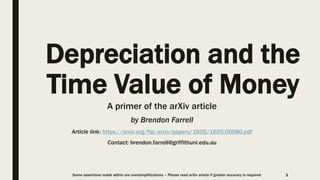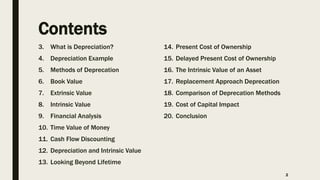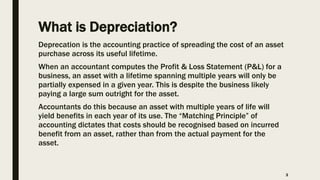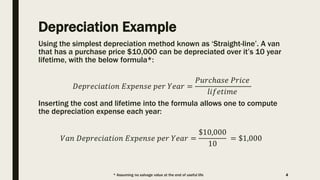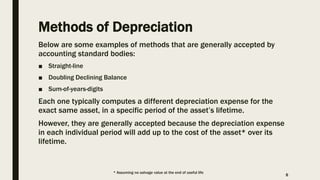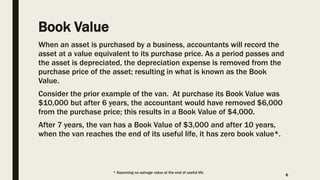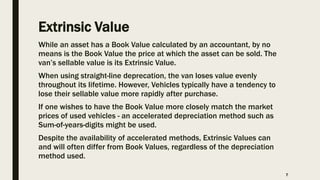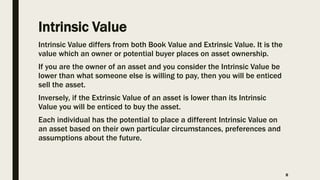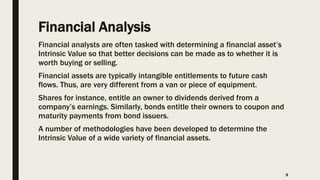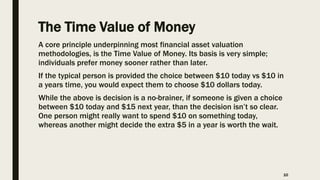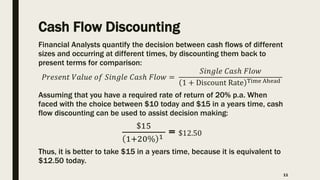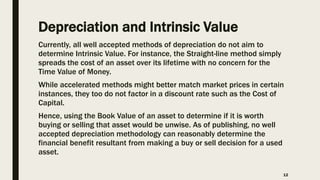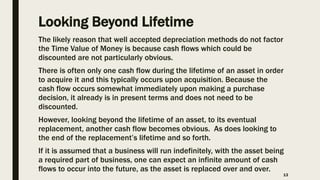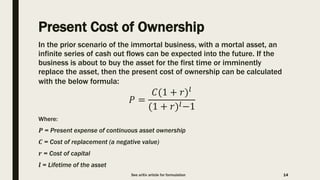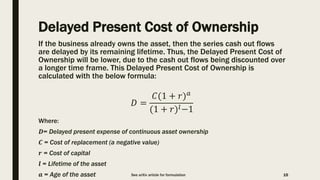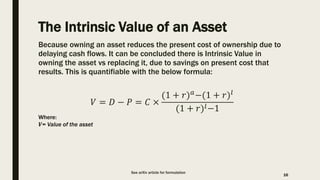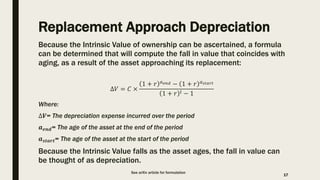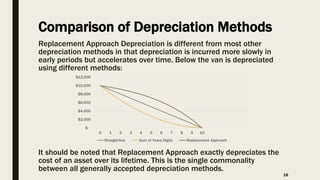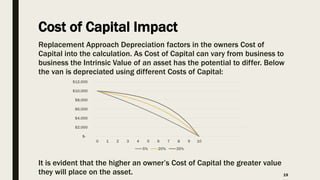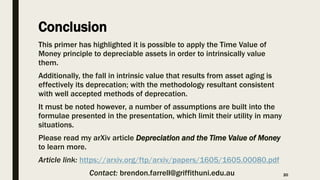1 sur 20
Publicité

### Depreciation and the Time Value of Money: A primer of the arXiv article

1. Depreciation and the Time Value of Money A primer of the arXiv article by Brendon Farrell Article link: https://arxiv.org/ftp/arxiv/papers/1605/1605.00080.pdf Contact: brendon.farrell@griffithuni.edu.au 1Some assertions made within are oversimplifications – Please read arXiv article if greater accuracy is required
2. Contents 3. What is Depreciation? 4. Depreciation Example 5. Methods of Deprecation 6. Book Value 7. Extrinsic Value 8. Intrinsic Value 9. Financial Analysis 10. Time Value of Money 11. Cash Flow Discounting 12. Depreciation and Intrinsic Value 13. Looking Beyond Lifetime 14. Present Cost of Ownership 15. Delayed Present Cost of Ownership 16. The Intrinsic Value of an Asset 17. Replacement Approach Deprecation 18. Comparison of Deprecation Methods 19. Cost of Capital Impact 20. Conclusion 2
3. What is Depreciation? Deprecation is the accounting practice of spreading the cost of an asset purchase across its useful lifetime. When an accountant computes the Profit & Loss Statement (P&L) for a business, an asset with a lifetime spanning multiple years will only be partially expensed in a given year. This is despite the business likely paying a large sum outright for the asset. Accountants do this because an asset with multiple years of life will yield benefits in each year of its use. The “Matching Principle” of accounting dictates that costs should be recognised based on incurred benefit from an asset, rather than from the actual payment for the asset. 3
4. Depreciation Example Using the simplest depreciation method known as ‘Straight-line’. A van that has a purchase price \$10,000 can be depreciated over it’s 10 year lifetime, with the below formula*: 𝐷𝑒𝑝𝑟𝑒𝑐𝑖𝑎𝑡𝑖𝑜𝑛 𝐸𝑥𝑝𝑒𝑛𝑠𝑒 𝑝𝑒𝑟 𝑌𝑒𝑎𝑟 = 𝑃𝑢𝑟𝑐ℎ𝑎𝑠𝑒 𝑃𝑟𝑖𝑐𝑒 𝑙𝑖𝑓𝑒𝑡𝑖𝑚𝑒 Inserting the cost and lifetime into the formula allows one to compute the depreciation expense each year: 𝑉𝑎𝑛 𝐷𝑒𝑝𝑟𝑒𝑐𝑖𝑎𝑡𝑖𝑜𝑛 𝐸𝑥𝑝𝑒𝑛𝑠𝑒 𝑝𝑒𝑟 𝑌𝑒𝑎𝑟 = \$10,000 10 = \$1,000 4* Assuming no salvage value at the end of useful life
5. Methods of Depreciation Below are some examples of methods that are generally accepted by accounting standard bodies: ■ Straight-line ■ Doubling Declining Balance ■ Sum-of-years-digits Each one typically computes a different depreciation expense for the exact same asset, in a specific period of the asset’s lifetime. However, they are generally accepted because the depreciation expense in each individual period will add up to the cost of the asset* over its lifetime. 5 * Assuming no salvage value at the end of useful life
6. Book Value When an asset is purchased by a business, accountants will record the asset at a value equivalent to its purchase price. As a period passes and the asset is depreciated, the depreciation expense is removed from the purchase price of the asset; resulting in what is known as the Book Value. Consider the prior example of the van. At purchase its Book Value was \$10,000 but after 6 years, the accountant would have removed \$6,000 from the purchase price; this results in a Book Value of \$4,000. After 7 years, the van has a Book Value of \$3,000 and after 10 years, when the van reaches the end of its useful life, it has zero book value*. 6 * Assuming no salvage value at the end of useful life
7. Extrinsic Value While an asset has a Book Value calculated by an accountant, by no means is the Book Value the price at which the asset can be sold. The van’s sellable value is its Extrinsic Value. When using straight-line deprecation, the van loses value evenly throughout its lifetime. However, Vehicles typically have a tendency to lose their sellable value more rapidly after purchase. If one wishes to have the Book Value more closely match the market prices of used vehicles - an accelerated depreciation method such as Sum-of-years-digits might be used. Despite the availability of accelerated methods, Extrinsic Values can and will often differ from Book Values, regardless of the depreciation method used. 7
8. Intrinsic Value Intrinsic Value differs from both Book Value and Extrinsic Value. It is the value which an owner or potential buyer places on asset ownership. If you are the owner of an asset and you consider the Intrinsic Value be lower than what someone else is willing to pay, then you will be enticed sell the asset. Inversely, if the Extrinsic Value of an asset is lower than its Intrinsic Value you will be enticed to buy the asset. Each individual has the potential to place a different Intrinsic Value on an asset based on their own particular circumstances, preferences and assumptions about the future. 8
9. Financial Analysis Financial analysts are often tasked with determining a financial asset’s Intrinsic Value so that better decisions can be made as to whether it is worth buying or selling. Financial assets are typically intangible entitlements to future cash flows. Thus, are very different from a van or piece of equipment. Shares for instance, entitle an owner to dividends derived from a company’s earnings. Similarly, bonds entitle their owners to coupon and maturity payments from bond issuers. A number of methodologies have been developed to determine the Intrinsic Value of a wide variety of financial assets. 9
10. The Time Value of Money A core principle underpinning most financial asset valuation methodologies, is the Time Value of Money. Its basis is very simple; individuals prefer money sooner rather than later. If the typical person is provided the choice between \$10 today vs \$10 in a years time, you would expect them to choose \$10 dollars today. While the above is decision is a no-brainer, if someone is given a choice between \$10 today and \$15 next year, than the decision isn’t so clear. One person might really want to spend \$10 on something today, whereas another might decide the extra \$5 in a year is worth the wait. 10
11. Cash Flow Discounting Financial Analysts quantify the decision between cash flows of different sizes and occurring at different times, by discounting them back to present terms for comparison: 𝑃𝑟𝑒𝑠𝑒𝑛𝑡 𝑉𝑎𝑙𝑢𝑒 𝑜𝑓 𝑆𝑖𝑛𝑔𝑙𝑒 𝐶𝑎𝑠ℎ 𝐹𝑙𝑜𝑤 = 𝑆𝑖𝑛𝑔𝑙𝑒 𝐶𝑎𝑠ℎ 𝐹𝑙𝑜𝑤 1 + Discount Rate Time Ahead Assuming that you have a required rate of return of 20% p.a. When faced with the choice between \$10 today and \$15 in a years time, cash flow discounting can be used to assist decision making: \$15 1+20% 1 = \$12.50 Thus, it is better to take \$15 in a years time, because it is equivalent to \$12.50 today. 11
12. Depreciation and Intrinsic Value Currently, all well accepted methods of depreciation do not aim to determine Intrinsic Value. For instance, the Straight-line method simply spreads the cost of an asset over its lifetime with no concern for the Time Value of Money. While accelerated methods might better match market prices in certain instances, they too do not factor in a discount rate such as the Cost of Capital. Hence, using the Book Value of an asset to determine if it is worth buying or selling that asset would be unwise. As of publishing, no well accepted depreciation methodology can reasonably determine the financial benefit resultant from making a buy or sell decision for a used asset. 12
13. Looking Beyond Lifetime The likely reason that well accepted depreciation methods do not factor the Time Value of Money is because cash flows which could be discounted are not particularly obvious. There is often only one cash flow during the lifetime of an asset in order to acquire it and this typically occurs upon acquisition. Because the cash flow occurs somewhat immediately upon making a purchase decision, it already is in present terms and does not need to be discounted. However, looking beyond the lifetime of an asset, to its eventual replacement, another cash flow becomes obvious. As does looking to the end of the replacement’s lifetime and so forth. If it is assumed that a business will run indefinitely, with the asset being a required part of business, one can expect an infinite amount of cash flows to occur into the future, as the asset is replaced over and over. 13
14. Present Cost of Ownership In the prior scenario of the immortal business, with a mortal asset, an infinite series of cash out flows can be expected into the future. If the business is about to buy the asset for the first time or imminently replace the asset, then the present cost of ownership can be calculated with the below formula: 𝑃 = 𝐶(1 + 𝑟)𝑙 (1 + 𝑟)𝑙−1 Where: 𝑷 = Present expense of continuous asset ownership 𝑪 = Cost of replacement (a negative value) 𝒓 = Cost of capital 𝒍 = Lifetime of the asset 14See arXiv article for formulation
15. Delayed Present Cost of Ownership If the business already owns the asset, then the series cash out flows are delayed by its remaining lifetime. Thus, the Delayed Present Cost of Ownership will be lower, due to the cash out flows being discounted over a longer time frame. This Delayed Present Cost of Ownership is calculated with the below formula: 𝐷 = 𝐶(1 + 𝑟) 𝑎 (1 + 𝑟)𝑙−1 Where: 𝑫= Delayed present expense of continuous asset ownership 𝑪 = Cost of replacement (a negative value) 𝒓 = Cost of capital 𝒍 = Lifetime of the asset 𝒂 = Age of the asset 15See arXiv article for formulation
16. The Intrinsic Value of an Asset Because owning an asset reduces the present cost of ownership due to delaying cash flows. It can be concluded there is Intrinsic Value in owning the asset vs replacing it, due to savings on present cost that results. This is quantifiable with the below formula: 𝑉 = 𝐷 − 𝑃 = 𝐶 × (1 + 𝑟) 𝑎−(1 + 𝑟)𝑙 (1 + 𝑟)𝑙−1 16 See arXiv article for formulation Where: 𝑽= Value of the asset
17. Replacement Approach Depreciation Because the Intrinsic Value of ownership can be ascertained, a formula can be determined that will compute the fall in value that coincides with aging, as a result of the asset approaching its replacement: ∆𝑉 = 𝐶 × 1 + 𝑟 𝑎 𝑒𝑛𝑑 − 1 + 𝑟 𝑎 𝑠𝑡𝑎𝑟𝑡 1 + 𝑟 𝑙 − 1 Where: ∆𝑽= The depreciation expense incurred over the period 𝒂 𝒆𝒏𝒅= The age of the asset at the end of the period 𝒂 𝒔𝒕𝒂𝒓𝒕= The age of the asset at the start of the period Because the Intrinsic Value falls as the asset ages, the fall in value can be thought of as depreciation. 17 See arXiv article for formulation
18. Comparison of Depreciation Methods Replacement Approach Depreciation is different from most other depreciation methods in that depreciation is incurred more slowly in early periods but accelerates over time. Below the van is depreciated using different methods: It should be noted that Replacement Approach exactly depreciates the cost of an asset over its lifetime. This is the single commonality between all generally accepted depreciation methods. 18 \$- \$2,000 \$4,000 \$6,000 \$8,000 \$10,000 \$12,000 0 1 2 3 4 5 6 7 8 9 10 Straight-line Sum of Years Digits Replacement Approach
19. Cost of Capital Impact Replacement Approach Depreciation factors in the owners Cost of Capital into the calculation. As Cost of Capital can vary from business to business the Intrinsic Value of an asset has the potential to differ. Below the van is depreciated using different Costs of Capital: It is evident that the higher an owner’s Cost of Capital the greater value they will place on the asset. 19 \$- \$2,000 \$4,000 \$6,000 \$8,000 \$10,000 \$12,000 0 1 2 3 4 5 6 7 8 9 10 5% 20% 35%
20. Conclusion This primer has highlighted it is possible to apply the Time Value of Money principle to depreciable assets in order to intrinsically value them. Additionally, the fall in intrinsic value that results from asset aging is effectively its deprecation; with the methodology resultant consistent with well accepted methods of deprecation. It must be noted however, a number of assumptions are built into the formulae presented in the presentation, which limit their utility in many situations. Please read my arXiv article Depreciation and the Time Value of Money to learn more. Article link: https://arxiv.org/ftp/arxiv/papers/1605/1605.00080.pdf Contact: brendon.farrell@griffithuni.edu.au 20
Publicité